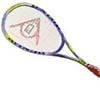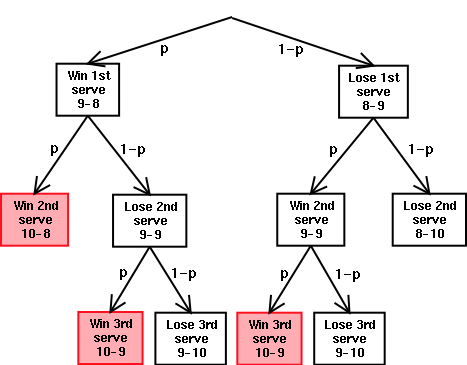#### You may also like### Rain or Shine

Predict future weather using the probability that tomorrow is wet given today is wet and the probability that tomorrow is wet given that today is dry.### Playing Squash

Playing squash involves lots of mathematics. This article explores the mathematics of a squash match and how a knowledge of probability could influence the choices you make.### Which Spinners?

Can you work out which spinners were used to generate the frequency charts?

# Squash

##### Age 16 to 18 Challenge Level:

In the game of squash, the player who serves is the only player who can win the next point. If the server loses the rally, no points are scored and the other player serves next. Note that if in two consecutive rallies both servers lose the rally, then the situation is exactly the same as it was before the two serves. This is not taken into account below where the tree diagram and solution from Allan Ling apply to the simpler problem where the player who wins a rally wins the point whether serving or receiving. Can you see how to re-draw the tree diagram to give the solution for the game of squash?

If I call '9', then I win with probability p.

If I call '10', then there are 6 possible outcomes, shown by the tree diagram.The winning outcomes are shown in red. Calculating the probabilities of these three outcomes, we find that the total is p 2 + p(1-p)p + (1-p)p 2 = 3p 2 - 2p .

Now we compare this to p to see which is larger.
When p< 0.5, then p> 3p 2 -2p 3 .
When p=0.5, then p=3p 2 -2p 3 .
When p> 0.5, then p< 3p 2 -2p 3 .

Therefore, in this simplified situation, if p is smaller than 0.5, I should call '9', and if p is greater than 0.5, I should call '10'. If p=0.5, I have an equal chance of winning on both choices.

Now what happens playing according to the rules of squash?

There is a detailed explanation of the solution to this problem in this article.